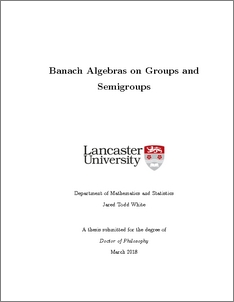# Banach algebras on groups and semigroups

White, Jared (2018) Banach algebras on groups and semigroups. PhD thesis, UNSPECIFIED.Preview
PDF (2018whitephd)
PhD_Thesis.pdf - Published Version

## Abstract

This thesis concerns the theory of Banach algebras, particularly those coming from abstract harmonic analysis. The focus for much of the thesis is the theory of the ideals of these algebras. In the final chapter we use semigroup algebras to solve an open probelm in the theory of C*-algebras. Throughout the thesis we are interested in the interplay between abstract algebra and analysis. Chapters 2, 4, and 5 are closely based upon the articles , , and , respectively. In Chapter 2 we study (algebraic) finite-generation of closed left ideals in Banach algebras. Let G be a locally compact group. We prove that the augmentation ideal in L 1 pGq is finitely-generated as a left ideal if and only if G is finite. We then investigate weighted versions of this result, as well as a version for semigroup algebras. Weighted measure algebras are also considered. We are motivated by a recent conjecture of Dales and Żelazko, which states that a unital Banach algebra in which every maximal left ideal is finitely-generated is necessarily finite-dimensional. We prove that this conjecture holds for many of the algebras considered. Finally, we use the theory that we have developed to construct some examples of commutative Banach algebras that relate to a theorem of Gleason. In Chapter 3 we turn our attention to topological finite-generation of closed left ideals in Banach algebras. We define a Banach algebra to be topologically left Noetherian if every closed left ideal is topologically finitely-generated, and we seek infinitedimensional examples of such algebras. We show that, given a compact group G, the group algebra L 1 pGq is topologically left Noetherian if and only if G is metrisable. For a Banach space E satisying a certain condition we show that the Banach algebra of approximable operators ApEq is topologically left Noetherian if and only if E 1 is separable, whereas it is topologically right Noetherian if and only if E is separable. We also define what it means for a dual Banach algebra to be weak*-topologically left Noetherian, and give examples which satisfy and fail this condition. Along the way, we give classifications of the weak*-closed left ideals in MpGq, for G a compact group, and in BpEq, for E a reflexive Banach space with AP. Chapter 4 looks at the Jacobson radical of the bidual of a Banach algebra. We prove that the bidual of a Beurling algebra on Z, considered as a Banach algebra with the first Arens product, can never be semisimple. We then show that rad p` 1 p‘8 i“1Zq 2 q contains nilpotent elements of every index. Each of these results settles a question of Dales and Lau. Finally we show that there exists a weight ω on Z such that the bidual of ` 1 pZ, ωq contains a radical element which is not nilpotent. In Chapter 5 we move away from the theory of ideals and consider a question about the notion of finiteness in C*-alegebras. We construct a unital pre-C*-algebra A0 which is stably finite, in the sense that every left invertible square matrix over A0 is right invertible, while the C*-completion of A0 contains a non-unitary isometry, and so it is infinite. This answers a question of Choi. The construction is based on semigroup algebras.

Item Type:
Thesis (PhD)
Departments:
ID Code:
125490
Deposited By:
Deposited On:
31 May 2018 08:46
Refereed?:
No
Published?:
Published